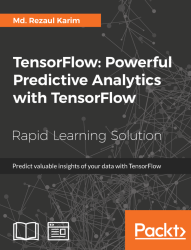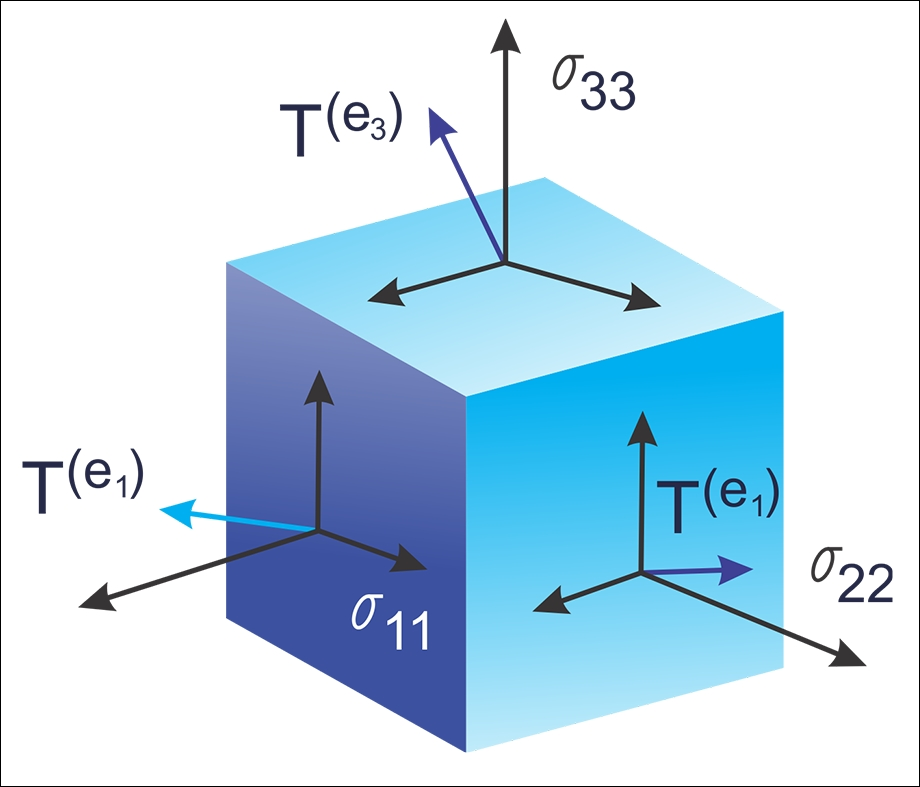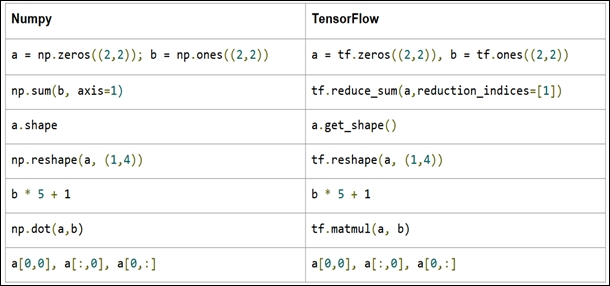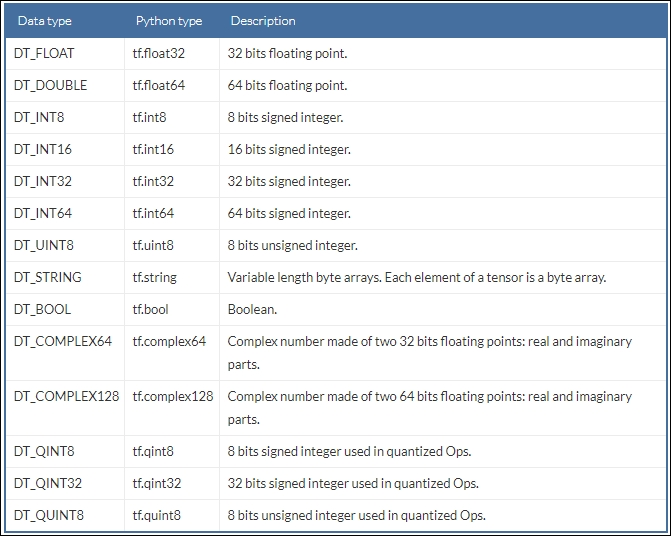•#### TensorFlow: Powerful Predictive Analytics with TensorFlow#### Overview of this book

Predictive analytics discovers hidden patterns from structured and unstructured data for automated decision making in business intelligence. Predictive decisions are becoming a huge trend worldwide, catering to wide industry sectors by predicting which decisions are more likely to give maximum results. TensorFlow, Google’s brainchild, is immensely popular and extensively used for predictive analysis. This book is a quick learning guide on all the three types of machine learning, that is, supervised, unsupervised, and reinforcement learning with TensorFlow. This book will teach you predictive analytics for high-dimensional and sequence data. In particular, you will learn the linear regression model for regression analysis. You will also learn how to use regression for predicting continuous values. You will learn supervised learning algorithms for predictive analytics. You will explore unsupervised learning and clustering using K-meansYou will then learn how to predict neighborhoods using K-means, and then, see another example of clustering audio clips based on their audio features. This book is ideal for developers, data analysts, machine learning practitioners, and deep learning enthusiasts who want to build powerful, robust, and accurate predictive models with the power of TensorFlow. This book is embedded with useful assessments that will help you revise the concepts you have learned in this book. This book is repurposed for this specific learning experience from material from Packt's Predictive Analytics with TensorFlow by Md. Rezaul Karim.
TensorFlow: Powerful Predictive Analytics with TensorFlowCreditsPrefaceFree Chapter
From Data to Decisions – Getting Started with TensorFlowPutting Data in Place – Supervised Learning for Predictive AnalyticsClustering Your Data – Unsupervised Learning for Predictive AnalyticsUsing Reinforcement Learning for Predictive Analytics## Data Model in TensorFlow

The data model in TensorFlow is represented by tensors. Without using complex mathematical definitions, we can say that a tensor (in TensorFlow) identifies a multidimensional numerical array. But we will see more details on tensor in the next sub-section.

### Tensors

Let's see a formal definition of tensors from Wikipedia (https://en.wikipedia.org/wiki/Tensor) as follows:

"Tensors are geometric objects that describe linear relations between geometric vectors, scalars, and other tensors. Elementary examples of such relations include the dot product, the cross product, and linear maps. Geometric vectors, often used in physics and engineering applications, and scalars themselves are also tensors."

This data structure is characterized by three parameters: Rank, Shape, and Type, as shown in the following figure:Figure 9: Tensors are nothing but geometrics objects having shape, rank, and type used to hold multidimensional arrays

A tensor thus can be thought of as a generalization of a matrix that specifies an element by an arbitrary number of indices. While practically used, the syntax for tensors is even more or less like nested vectors.

### Note

Tensors just define the type of this value and the means by which this value should be calculated during the session. Therefore, essentially, they do not represent or hold any value produced by an operation.

A few people love to compare NumPy versus TensorFlow comparison; however, in reality, TensorFlow and NumPy are quite similar in a sense that both are N-d array libraries!

Well, it's true that NumPy has the n–dimensional array support, but it doesn't offer methods to create tensor functions and automatically compute derivatives (+ no GPU support). The following table can be seen as a short and one-to-one comparison that could make some sense of such comparisons:Figure 10: NumPy versus TensorFlow

Now let's see an alternative way of creating tensors before they could be fed (we will see other feeding mechanisms later on) by the TensorFlow graph:

```>>> X = [[2.0, 4.0],
[6.0, 8.0]]
>>> Y = np.array([[2.0, 4.0],
[6.0, 6.0]], dtype=np.float32)
>>> Z = tf.constant([[2.0, 4.0],
[6.0, 8.0]])```

Here `X` is a list, `Y` is an n-dimensional array from the NumPy library, and `Z` is itself the TensorFlow's Tensor object. Now let's see their types:

```>>> print(type(X))
>>> print(type(Y))
>>> print(type(Z))
#Output
<class 'list'>
<class 'numpy.ndarray'>
<class 'tensorflow.python.framework.ops.Tensor'>```

Well, their types are printed correctly. However, a more convenient function that we're formally dealing with tensors, as opposed to the other types is `tf.convert_to_tensor()` function as follows:

`t1 = tf.convert_to_tensor(X, dtype=tf.float32)t2 = tf.convert_to_tensor(Z, dtype=tf.float32)t3 = tf.convert_to_tensor(Z, dtype=tf.float32)`

Now let's see their type using the following lines:

```>>> print(type(t1))
>>> print(type(t2))
>>> print(type(t3))
#Output:
<class 'tensorflow.python.framework.ops.Tensor'>
<class 'tensorflow.python.framework.ops.Tensor'>
<class 'tensorflow.python.framework.ops.Tensor'>```

Fantastic! I think up to now it's enough discussion already carried out on tensors, so now we can think about the structure that is characterized by the term rank.

### Rank

Each tensor is described by a unit of dimensionality called rank. It identifies the number of dimensions of the tensor, for this reason, a rank is known as order or n–dimensions of a tensor. A rank zero tensor is a scalar, a rank one tensor id a vector, while a rank two tensor is a matrix. The following code defines a TensorFlow scalar, a `vector`, a `matrix`, and a `cube_matrix`, in the next example we will show how the rank works:

```import tensorflow as tf
scalar = tf.constant(100)
vector = tf.constant([1,2,3,4,5])
matrix = tf.constant([[1,2,3],[4,5,6]])
cube_matrix = tf.constant([[,,],[,,],[,,]])
print(scalar.get_shape())
print(vector.get_shape())
print(matrix.get_shape())
print(cube_matrix.get_shape())```

The results are printed here:

```>>>
()
(5,)
(2, 3)
(3, 3, 1)
>>>```

### Shape

The shape of a tensor is the number of rows and columns it has. Now we will see how to relate the shape to a rank of a tensor:

```>>scalar1.get_shape()
TensorShape([])
>>vector1.get_shape()
TensorShape([Dimension(5)])
>>matrix1.get_shape()
TensorShape([Dimension(2), Dimension(3)])
>>cube1.get_shape()
TensorShape([Dimension(3), Dimension(3), Dimension(1)])```

### Data Type

In addition to rank and shape, tensors have a data type. The following is the list of the data types:We believe the preceding table is self-explanatory hence we did not provide detailed discussion on the preceding data types. Now the TensorFlow APIs are implemented to manage data to and from NumPy arrays. Thus, to build a tensor with a constant value, pass a NumPy array to the `tf.constant()` operator, and the result will be a TensorFlow tensor with that value:

```import tensorflow as tf
import numpy as np
tensor_1d = np.array([1,2,3,4,5,6,7,8,9,10])
tensor_1d = tf.constant(tensor_1d)
with tf.Session() as sess:
print (tensor_1d.get_shape())
print sess.run(tensor_1d)
# Finally, close the TensorFlow session when you're done
sess.close()```

Running the example, we obtain:

```>>>
(10,)
[ 1  2  3  4  5  6  7  8  9 10]```

To build a tensor, with variable values, use a `NumPy` array and pass it to the `tf.Variable `constructor, the result will be a TensorFlow variable tensor with that initial value:

```import tensorflow as tf
import numpy as np
tensor_2d = np.array([(1,2,3),(4,5,6),(7,8,9)])
tensor_2d = tf.Variable(tensor_2d)
with tf.Session() as sess:
sess.run(tf.global_variables_initializer())
print (tensor_2d.get_shape())
print sess.run(tensor_2d)
# Finally, close the TensorFlow session when you're done
sess.close()```

The result is:

```>>>
(3, 3)
[[1 2 3]
[4 5 6]
[7 8 9]]```

For ease of use in interactive Python environments, we can use the `InteractiveSession` class, and then use that session for all `Tensor.eval() `and `Operation.run() `calls:

```import tensorflow as tf
import numpy as np

interactive_session = tf.InteractiveSession()
tensor = np.array([1,2,3,4,5])
tensor = tf.constant(tensor)
print(tensor.eval())

interactive_session.close()```

### Note

`tf.InteractiveSession()` is just a convenient syntactic sugar for keeping a default session open in IPython.

The result is:

```>>>
[1 2 3 4 5]```

This can be easier in an interactive setting, such as the shell or an IPython notebook, when it's tedious to pass around a session object everywhere.

### Note

The IPython Notebook is now known as the Jupyter Notebook. It is an interactive computational environment, in which you can combine code execution, rich text, mathematics, plots and rich media. For more information, interested readers should refer to the web page at https://ipython.org/notebook.html.

Another way to define a tensor is using the TensorFlow statement `tf.convert_to_tensor`:

```import tensorflow as tf
import numpy as np
tensor_3d = np.array([[[0, 1, 2], [3, 4, 5], [6, 7, 8]],
[[9, 10, 11], [12, 13, 14], [15, 16, 17]],
[[18, 19, 20], [21, 22, 23], [24, 25, 26]]])
tensor_3d = tf.convert_to_tensor(tensor_3d, dtype=tf.float64)
with tf.Session() as sess:
print(tensor_3d.get_shape())
print(sess.run(tensor_3d))
# Finally, close the TensorFlow session when you're done
sess.close()
>>>
(3, 3, 3)
[[[  0.   1.   2.]
[  3.   4.   5.]
[  6.   7.   8.]]
[[  9.  10.  11.]
[ 12.  13.  14.]
[ 15.  16.  17.]]
[[ 18.  19.  20.]
[ 21.  22.  23.]
[ 24.  25.  26.]]]```

### Variables

Variables are TensorFlow objects to hold and update parameters. A variable must be initialized; also you can save and restore it to analyze your code. Variables are created by using the `tf.Variable() `statement. In the following example, we want to count the numbers from 1 to 10, but let's import TensorFlow first:

`import tensorflow as tf`

We created a variable that will be initialized to the scalar value `0`:

`value = tf.Variable(0, name="value")`

The `assign() `and `add()`operators are just nodes of the computation graph, so they do not execute the assignment until the run of the session:

```one = tf.constant(1)
update_value = tf.assign(value, new_value)
initialize_var = tf.global_variables_initializer()```

We can instantiate the computation graph:

```with tf.Session() as sess:
sess.run(initialize_var)
print(sess.run(value))
for _ in range(5):
sess.run(update_value)
print(sess.run(value))
# Finally, close the TensorFlow session when you're done:
sess.close()```

Let's recall that a tensor object is a symbolic handle to the result of an operation, but it does not actually hold the values of the operation's output:

```>>>
0
1
2
3
4
5```

### Fetches

To fetch the outputs of operations, execute the graph by calling `run()` on the session object and pass in the tensors to retrieve. Except fetching the single tensor node, you can also fetch multiple tensors. In the following example, the sum and multiply tensors are fetched together, using the `run()` call:

```import tensorflow as tf

constant_A = tf.constant([100.0])
constant_B = tf.constant([300.0])
constant_C = tf.constant([3.0])

mul_ = tf.multiply(constant_A,constant_C)

with tf.Session() as sess:
result = sess.run([sum_,mul_])
print(result)

# Finally, close the TensorFlow session when you're done:
sess.close()```

The output is as follows:

```>>>
[array(400.],dtype=float32),array([ 300.],dtype=float32)]```

All the ops needed to produce the values of the requested tensors are run once (not once per requested tensor).

### Feeds and Placeholders

There are four methods of getting data into a TensorFlow program (see details at https://www.tensorflow.org/api_guides/python/reading_data):

• The Dataset API: This enables you to build complex input pipelines from simple and reusable pieces from distributed file systems and perform complex operations. Using the Dataset API is recommended while dealing with large amounts of data in different data formats. The Dataset API introduces two new abstractions to TensorFlow for creating feedable dataset using either `tf.contrib.data.Dataset` (by creating a source or applying a transformation operations) or using a `tf.contrib.data.Iterator`.

• Feeding: Allows us to inject data into any Tensor in a computation graph.

• Reading from files: We can develop an input pipeline using Python's built-in mechanism for reading data from data files at the beginning of a TensorFlow graph.

• Preloaded data: For small datasets, we can use either constants or variables in the TensorFlow graph for holding all the data.

In this section, we will see an example of the feeding mechanism only. For the other methods, we will see them in upcoming lesson. TensorFlow provides the feed mechanism that allows us inject data into any tensor in a computation graph. You can provide the feed data through the `feed_dict` argument to a `run()` or `eval()`invoke that initiates the computation.

### Note

Feeding using the `feed_dict` argument is the least efficient way to feed data into a TensorFlow execution graph and should only be used for small experiments needing small datasets. It can also be used for debugging.

We can also replace any tensor with feed data (that is variables and constants), the best practice is to use a TensorFlow placeholder node using `tf.placeholder()` invocation. A placeholder exists exclusively to serve as the target of feeds. An empty placeholder is not initialized so it does not contain any data. Therefore, it will always generate an error if it is executed without a feed, so you won't forget to feed it.

The following example shows how to feed data to build a random 2×3 matrix:

```import tensorflow as tf
import numpy as np

a = 3
b = 2
x = tf.placeholder(tf.float32,shape=(a,b))

data = np.random.rand(a,b)
sess = tf.Session()
print sess.run(y,feed_dict={x:data})

# Finally, close the TensorFlow session when you're done:
sess.close()```

The output is:

```>>>
[[ 1.78602004  1.64606333]
[ 1.03966308  0.99269408]
[ 0.98822606  1.50157797]]
>>>```